# How to Move Every Other Row to a New Column in Excel

This post will guide you how to move every other row to a new column in Excel. How do I move every odd row to another column with formula in Excel 2013/2016. How to move every even row to other column in Excel with VBA Macro in Excel.

Assuming that you have a list of data in range B1:B6, and you want to move every odd or even rows in range B1:B6 to another new column in your worksheet. How to do it. This post will show you two methods to accomplish it.

## Move Every Other Row to New Column with Formula

If you want to move every even row in range B1:B6 to another column, such as: Column C, just type the following formula based on the IF function, the ISEVEN function and the Row function in Cell C1. And then press Enter key to apply the formula. Drag the AutoFill handle down to other cells.

`=IF(ISEVEN(ROW(B1)),B1,"")`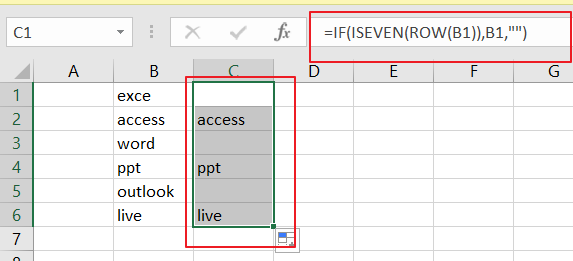If you want to move every odd row in range B1:B6 to another column, such as: Column D, just type the following formula in cell D1:

`=IF(ISODD(ROW(B1)),B1,"")`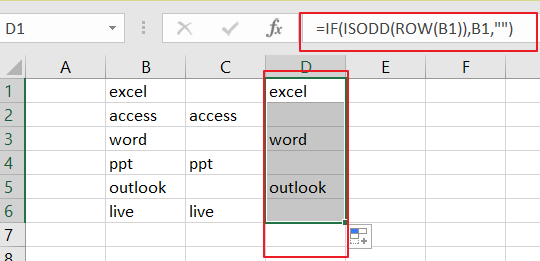## Move Every Other Row to New Column with VBA

You can also use an Excel VBA Macro to accomplish the same result of moving every other row to new columns. Just do the following steps:

Step1: open your excel workbook and then click on “Visual Basic” command under DEVELOPER Tab, or just press “ALT+F11” shortcut.

Step2: then the “Visual Basic Editor” window will appear.

Step3: click “Insert” ->”Module” to create a new module.

Step4: paste the below VBA code  into the code window. Then clicking “Save” button.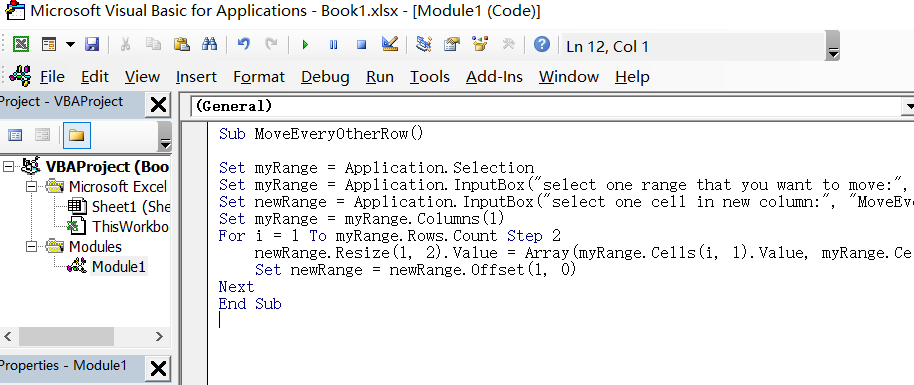```[vb]

Sub MoveEveryOtherRow()

Set myRange = Application.Selection
Set myRange = Application.InputBox("select one range that you want to move:", "MoveEveryOtherRow", myRange.Address, Type:=8)
Set newRange = Application.InputBox("select one cell in new column:", "MoveEveryOtherRow", Type:=8)
Set myRange = myRange.Columns(1)
For i = 1 To myRange.Rows.Count Step 2
newRange.Resize(1, 2).Value = Array(myRange.Cells(i, 1).Value, myRange.Cells(i + 1, 1).Value)
Set newRange = newRange.Offset(1, 0)
Next
End Sub

[/vb]```

Step5: back to the current worksheet, then run the above excel macro. Click Run button.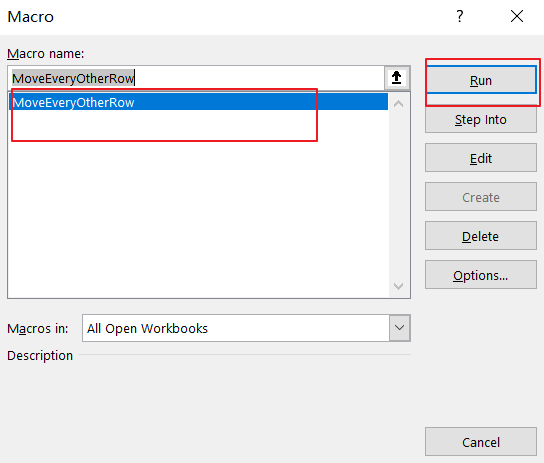Step6: select one range that you want to move. Click Ok button.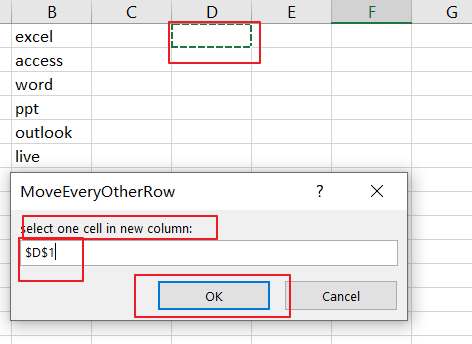Step7: select one cell in new column. Click Ok button.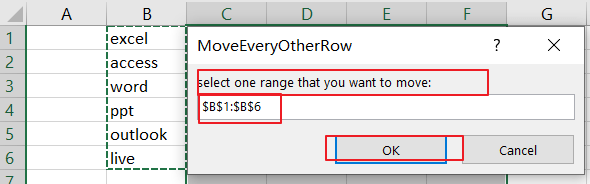Step8: let’s see the last result: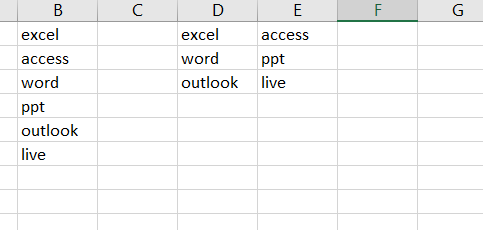### Related Functions

• Excel ROW function The Excel ROW function returns the row number of a cell reference.The ROW function is a build-in function in Microsoft Excel and it is categorized as a Lookup and Reference Function.The syntax of the ROW function is as below:= ROW ([reference])….
• Excel IF function The Excel IF function perform a logical test to return one value if the condition is TRUE and return another value if the condition is FALSE. The IF function is a build-in function in Microsoft Excel and it is categorized as a Logical Function.The syntax of the IF function is as below:= IF (condition, [true_value], [false_value])….